Series of ENGLISH MEDIUM Books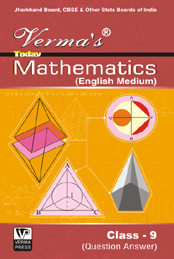Mathematics (English Medium)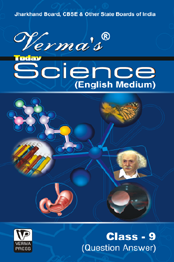Science (English Medium)Social Science (English Medium) Rs. 130.00 Rs. 130.00 Rs. 130.00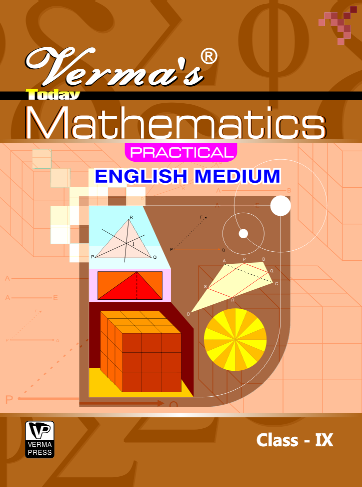Mathematics Practical (English Medium)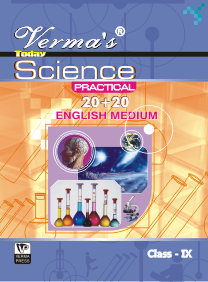Science Practical (English Medium)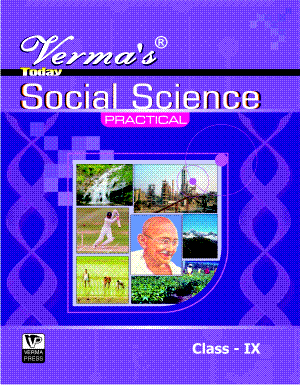Social Science Practical (English Medium) Rs. 60.00 Rs. 60.00 Rs. 70.00

Series of Hindi Medium Books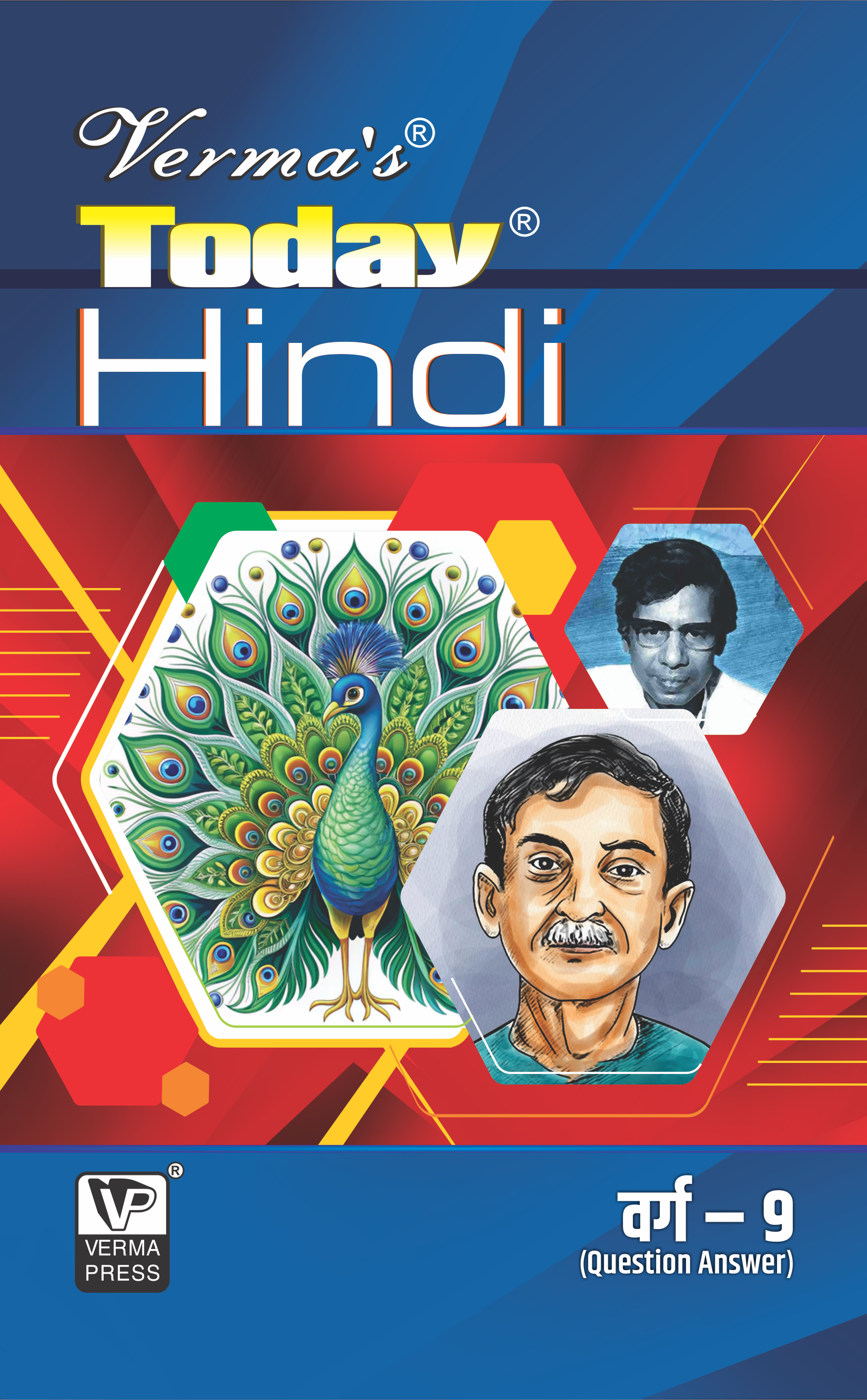HindiEnglishMathematics Rs. 100.00 Rs. 100.00 Rs. 100.00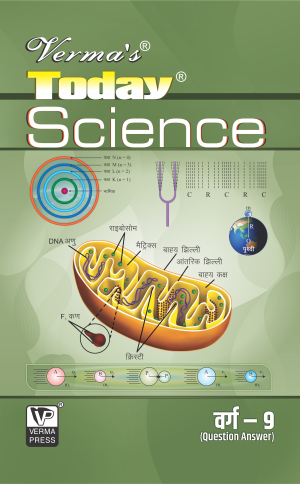Science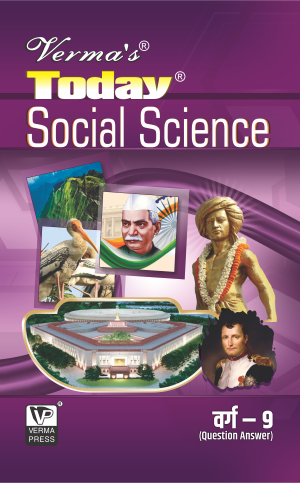Social ScienceSanskrit Rs. 100.00 Rs. 100.00 Rs. 100.00Hindi-B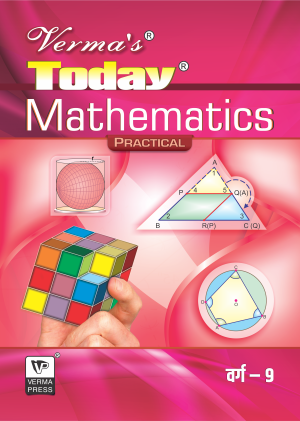Mathematics Practical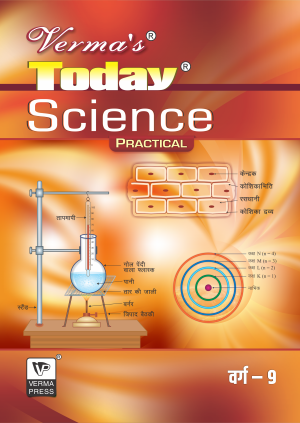Science Practical Rs. 80.00 Rs. 50.00 Rs. 50.00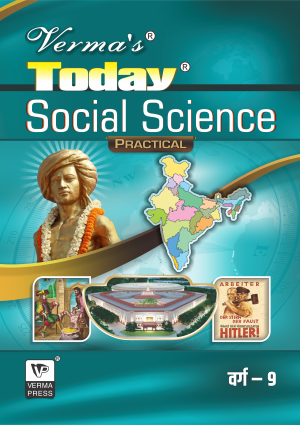Social Science PracticalMathematics PracticalScience Practical Rs. 50.00 Rs. 35.00 Rs. 35.00

MONTHLY TEST PAPER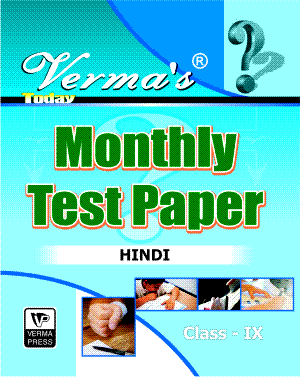HindiEnglishMathematics Rs. 80.00 Rs. 100.00 Rs. 100.00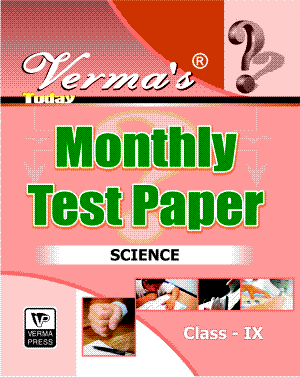Science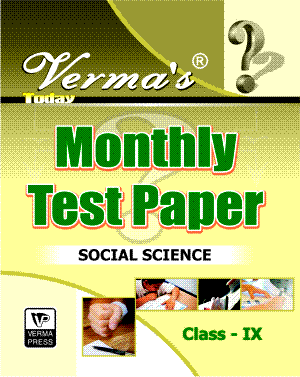Social Science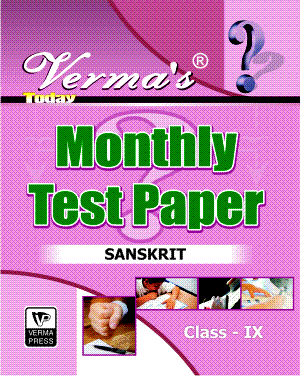Sanskrit Rs. 100.00 Rs. 100.00 Rs. 80.00

JHARKHAND ANNUAL TODAY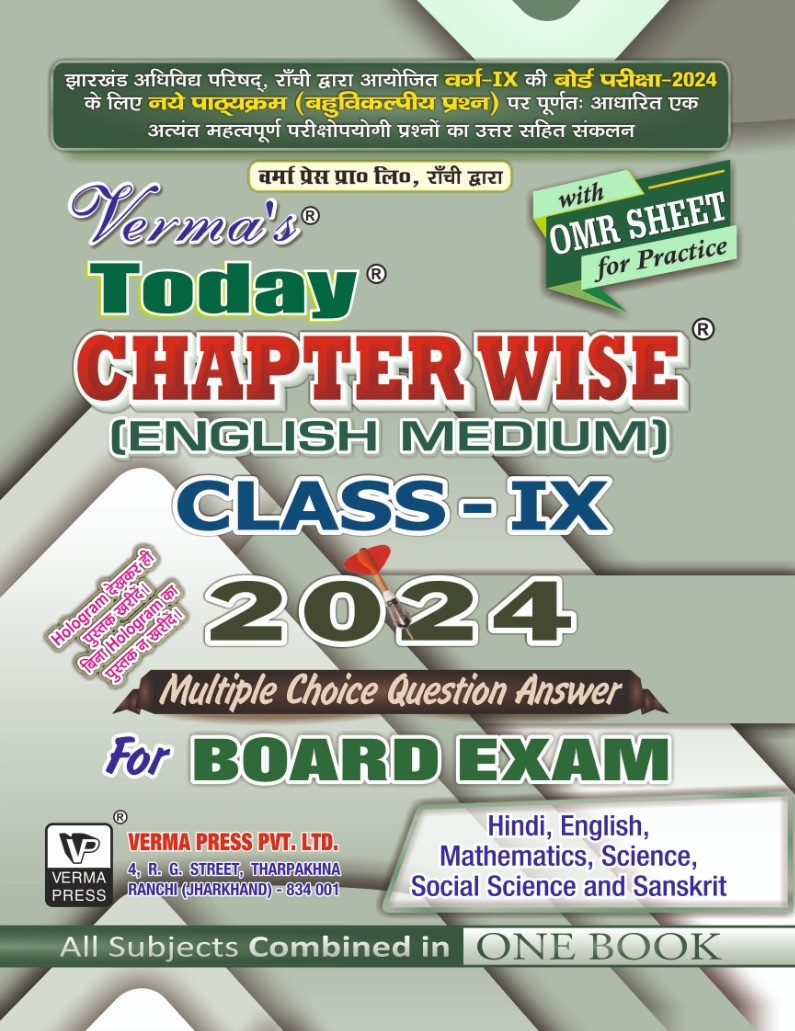Jharkhand Annual Today Rs. 70.00